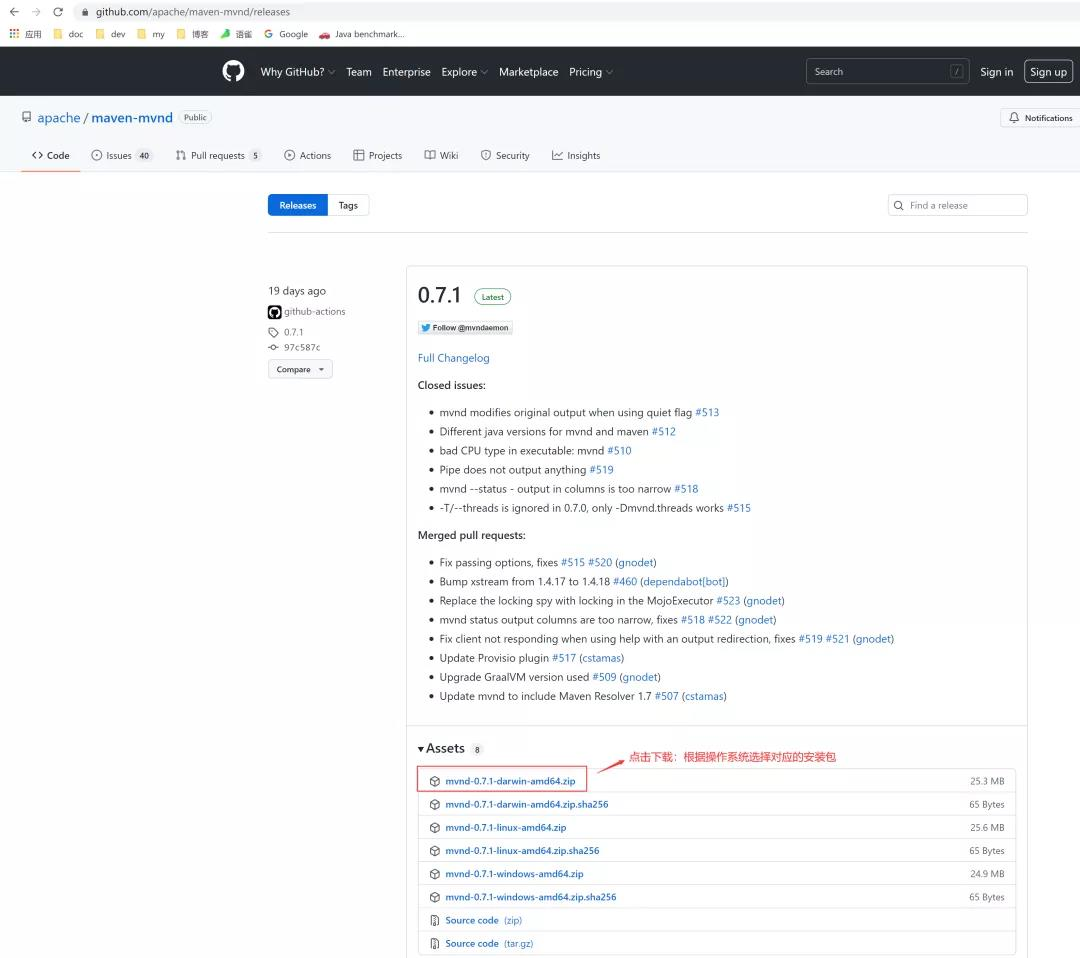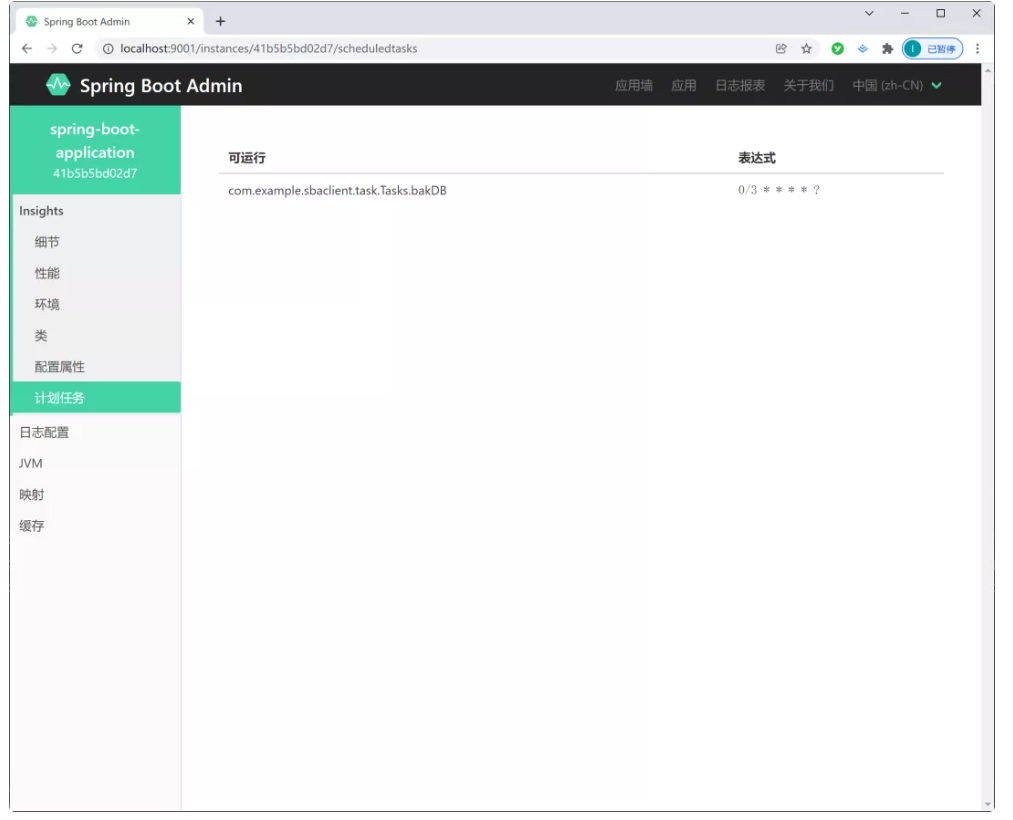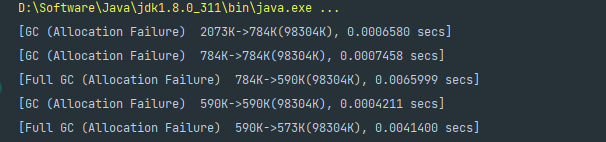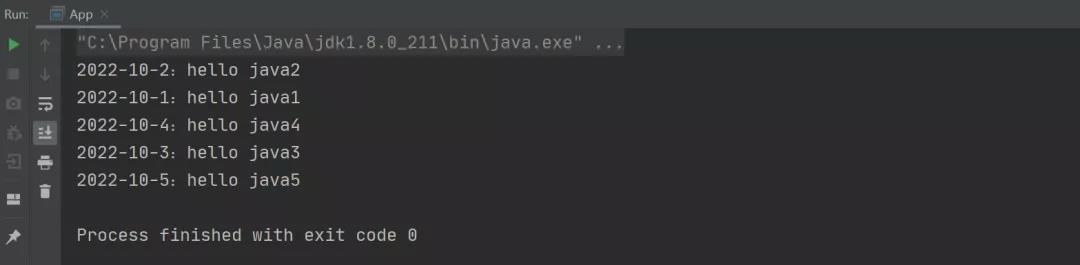【JavaScript框架封装】实现一个类似于JQuery的基础框架、事件框架、CSS框架、属性框架、内容框架、动画框架整体架构的搭建

+关注继续查看

/*
* @Author: 我爱科技论坛
* @Time: 20180715
* @Desc: 实现一个类似于JQuery功能的框架
* V 1.0: 实现了基础框架、事件框架、CSS框架、属性框架、内容框架、动画框架的搭建
* V 2.0：实现了框架的进一步优化，具有良好的扩展性， 可以支持链式访问
* */

/ 主框架: 只做一件事，就是用于获取所有的元素集合
(function (w) {
// 定义一个Xframe对象，后面就是他的构造函数
var xframe = function (selector, context) {
// 为了使得后面的函数this始终指向的是xframe框架，这里需要修改函数内部this的指向
return this.init.apply(this, [selector, context]);
};

// 定义一个初始化函数，用于初始化获取所有的元素集合
// 只要用户使用了类似于JQuery中的选择元素的方法，就开始选择一个元素集合
// 这里的核心功能：实际上是为了使用一个伪数组实现一个类似于JQuery中的链式访问的功能
xframe.prototype.init = function (selector, context) {
// 开始构建一个伪数组：{1 : list, 2 : list, , , , length : list.length}
this.length = 0;
if (typeof  selector === 'string'){
var nodeList = (context || document).querySelectorAll(selector);
this.length = nodeList.length;
for (var i = 0, len = this.length; i < len; i++){
this[i] = nodeList[i];
}
} else if (selector.nodeType){
// 如果获取的是一个元素节点，文本节点，或者属性节点的话
this = selector;
this.length++;
}

// 为了可以支持链式访问必须把这个this对象返回出去
return this;
};

// 使用双对象法则继续暴露出去一个对象，进行对象的二次封装
// 【双对象法则的使用】
var $$= function (selctor, context) { // 这里使用一个简单的异步加载机制，等待所有的DOM元素执行完毕之后再开始继续向下执行 if (typeof selctor === 'function'){ // selector就是DOM元素加载完毕之后的继续向下执行的回调函数 w.onload = selctor; } else { // 如果不是一个函数的话 return new xframe(selecor, context); } } // 添加一个extend方法， 用于扩充一个对象的方法， 扩展向一个类中拷贝方法$$.extend = function () {
// 这里需要分为两种情况：
// 1. 如果传过来的是一个参数的话，就相当于是给xframe对象添加方法
// 2. 如果是两个参数的话，就相当于是给一个类扩充方法
var len = arguments.length,
target = null,              // target 用来存储需要把方法拷贝进去的目标函数
i = 1,                      // 初始化变量i, 表示需要开始遍历的起始位置标记
key;                        // 为了防止定义太多的局部变量，可以把后面需要用到的所有局部变量事先在前面定义好
if (len === 0){
return;
} else if (len === 1){
// 给xrame对象添加方法
target = xframe.prototype;
i--;
} else{
// 两个参数的话，那么第一个参数就是我需要拷贝新的方法进去的目标对象
// 如果是两个参数的话：就不需要修改变量i的值了， 直接从第一个位置开始，拿到第一个参数后， 把第二个参数的方法全部拷贝给第一个对象
// 注意: 这里有可能也是三个参数或者是多个参数， 因此也可以吧后面的好几个对象的属性或者方法添加给第一个对象
target = arguments;
}

// 确定好了target 这个目标对象以后，开始遍历原始对象那个source，把source里面的方法全部都拷贝到这个target对象里面
for (; i < len; i++){
// 这里实际上在遍历一个json对象，json对象的每一项实际上就是一个属性或者方法
for (key in source){
target[key] = arguments[i][key];
}
}
return target;
}

// 为了把主框架里面的局部变量暴露出去供其他模块使用
w.xframe = w.$= $$; })(window); // 公共框架 (function (xframe) { // 需要参与链式访问的(必须使用prototype的方式来给对象扩充方法) xframe.extend({ each : function () { } }); // 不需要参与链式访问的 /*公共部分*/ xframe.extend(xframe, { }); /*字符串处理模块*/ xframe.extend(xframe, { }); /*数组相关*/ xframe.extend(xframe, { }); /*Math*/ xframe.extend(xframe, { }); /*数据类型检验*/ xframe.extend(xframe, { }); })(xframe); // 事件框架 (function (xframe) { // 需要参与链式访问的(必须使用prototype的方式来给对象扩充方法) xframe.extend({ each : function () { } }); // 不需要参与链式访问的 xframe.extend(xframe, { }); })(xframe); // 属性框架 (function (xframe) { // 需要参与链式访问的(必须使用prototype的方式来给对象扩充方法) xframe.extend({ each : function () { } }); // 不需要参与链式访问的 xframe.extend(xframe, { }); })(xframe); // 内容框架 (function (xframe) { // 需要参与链式访问的(必须使用prototype的方式来给对象扩充方法) xframe.extend({ each : function () { } }); // 不需要参与链式访问的 xframe.extend(xframe, { }); })(xframe); // DOM框架 (function (xframe) { // 需要参与链式访问的(必须使用prototype的方式来给对象扩充方法) xframe.extend({ each : function () { } }); // 不需要参与链式访问的 xframe.extend(xframe, { }); })(xframe); // 缓存框架 (function (xframe) { // 需要参与链式访问的(必须使用prototype的方式来给对象扩充方法) xframe.extend({ each : function () { } }); // 不需要参与链式访问的 xframe.extend(xframe, { }); })(xframe); // 基础框架: 基本上都是不需要参与链式访问的 // 实现功能: 如果是一个参数的话，就直接给类添加方法 // 如果是多个参数的话，就表示给指定的对象添加方法 xframe.extend(xframe, { ltrim: function () { }, rtrim: function () { }, trim: function () { }, ajax: function () { }, formateString: function () { }, random: function () { }, isNumber: function () { }, isBoolean: function () { }, isString: function () { }, isUndefined: function () { }, isObject: function () { }, isNull: function () { }, isArray: function () { }, }) // 选择框架 /*** * 如果下面的框架需要用到上面的框架，就直接可以把上面的去全局变量/对象暴露出去，这里是使用了前面的xframe全局变量 */ (function ($$) { $$.all = function () { };$$.$id = function (id) {

};
$$.tag = function () { };$$.\$class = function () {

};
$$.group = function () { };$$.cengci = function () {

};
$$.select = function () { } })(xframe); // 事件框架 (function ($$) {
$$.on = function () { } })(xframe); // CSS框架 (function ($$) {
$$.css = function () { }; // 元素的显示与隐藏$$.show = function () {

};
$$.hide = function () { }; // 元素的宽度和高度$$.width = function () {

};
$$.height = function () { }; // 元素的滚动宽度和高度$$.scrollWidth = function () {

};
$$.scrollHeight = function () { }; // 滚动条相对于上面和左边的距离$$.scrollTop = function () {

};
$$.scrollLeft = function () { }; // 屏幕的宽度和高度$$.screenWidth = function () {

};
$$.screenHeight = function () { }; // 文档区域的宽度和高度$$.wWidth = function () {

};
$$.wHeight = function () { }; // 获取文档滚动窗口的相关属性参数信息$$.wScrollHeight = function () {

};
$$.wScrollWidth = function () { }; // 获取文档滚动窗口距离上面和左边的距离和宽度$$.wScrollTop = function () {

};
$$.wScrollLeft = function () { }; })(xframe); // 属性框架 (function ($$) {
// 私有方法
function removeName() {

};

}

// 公有方法
$$.attr = function () { };$$.addClass = function () {

};
$$.removeClass = function () { } })(xframe); // 内容框架 (function () {$$.html = function () {

};
$$.text = function () { };$$.val = function () {

}
})();

// 动画框架
(function () {
function Animate() {

}

Animate.prototype = {}
})();45 0Gear的技术优势
Gear 关键的技术创新在于其新颖的跨合约通信方式。Gear 使用 Actor 通信模型和 WebAssembly VM，支持并行处理，并具有速度快、成本低的优势。 事实证明， WebAssembly VM 比任何其他方案运行速度都要快。使用 WebAssembly 可以让 GEAR 的智能合约直接编译成机器码，运行速度媲美原生。更快的速度意味着更低的交易成本和更高的效率。
9 027 0Chrome_Browse

10 0C 语言项目_项目流程|学习笔记

6 0mysql事务隔离级别
mysql事务隔离级别
11 0mysql使用问题定位
serverTimezone问题
6 0JVM深入学习(二十四)-GC日志
GC日志是分析垃圾回收不可缺少的工具,我们通过对GC日志的分析可以看到垃圾回收的详细过程,更好的调优垃圾回收
4 0HashMap 中的一个“坑”！（2）
HashMap 中的一个“坑”！（2）
35 0
53

0

《2021云上架构与运维峰会演讲合集》

《零基础CSS入门教程》

《零基础HTML入门教程》